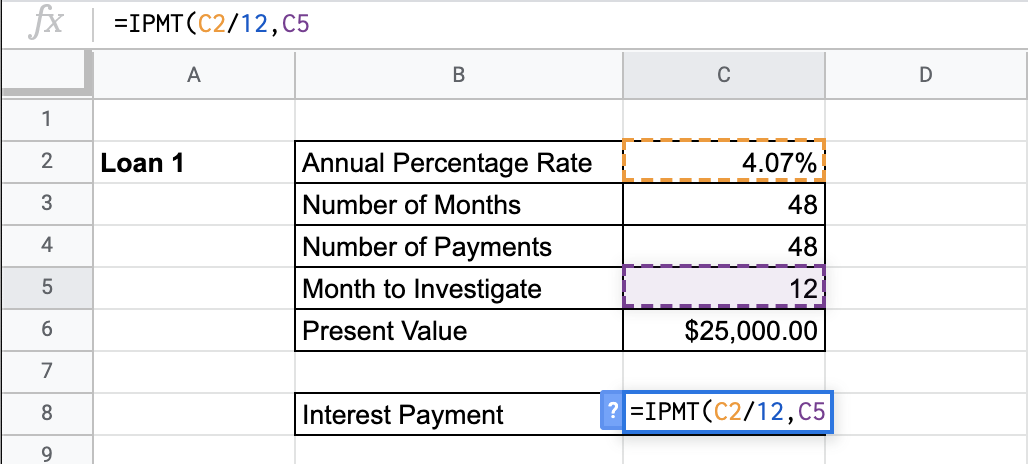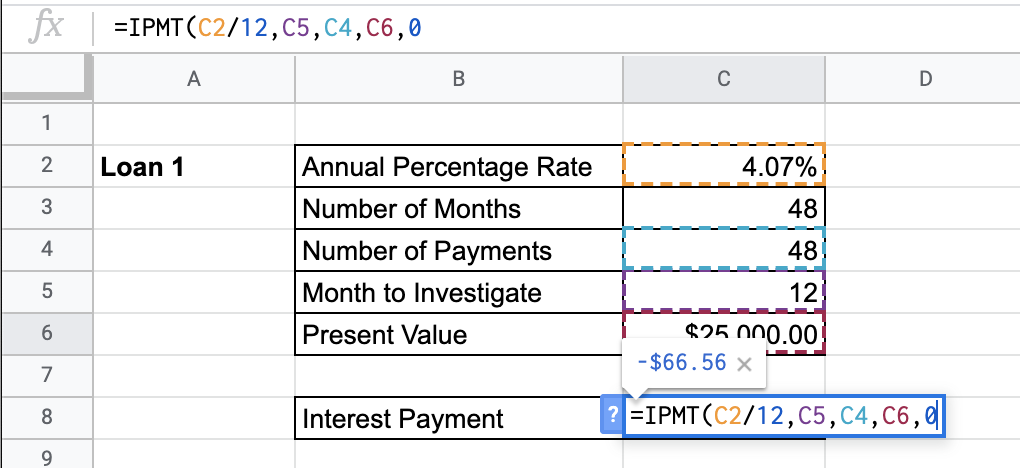# How to Use the IPMT Function in Google Sheets

The `IPMT` function in Google Sheets is used to calculate the payment on the interest for an investment based on constant-amount periodic payments and a constant interest rate.

When we calculate for the `PMT` function and find the correct payment periodic payment amount, this amount can be broken down to the principal repayment and the interest payment.

The `IPMT` function is useful because it helps you understand which part of a certain payment goes towards the interest. Having this information can help you plan around your investments and reach your investment goals in the proper manner. It is an easy, simplified method to help in your financial analysis problems.

Let’s look at an example.

You want to choose what investment to pursue, and you are planning your liquidity during certain periods. You have one upcoming month in mind. You have the information about the cost of the investment, the number of periods that the payment will take, and the fixed interest rates.

The `IPMT` function needs all the parameters mentioned above to help calculate the right interest payment for the investment in a certain period.

## The Anatomy of the IPMT Function in Google Sheets

The syntax of the `IPMT` function is as follows::

`=IPMT(rate, period, number_of_periods, present_value, [future_value,end_or_beginning]))`

Let’s have a look at each part of the function to understand what is going on here:

• `=` is the equals sign that starts off any function in Google Sheets.
• `IPMT` is the name of our function.
• `rate` is the interest rate.
• `period` is the amortization period, in terms of the number of periods.
• `number_of_periods` is the number of payments to be made.
• `present_value` is the current value of the annuity.
• `future_value` is an optional attribute that is the future value remaining after the final payment has been made.
• `end_or_beginning` is an optional attribute that sets whether the payments are due at the end (0) or the beginning (1) of each period. If left blank, it will be 0 by default.

You should also make sure that consistent units are used for the `rate` and `number_of_periods`. For example, if you have a loan, paid monthly, that goes on for longer than 12 months, and you have the annual percentage rate on hand, you need to divide this rate by 12. In the same vein, a loan paid quarterly requires you to divide the provided annual percentage rate by 4.

## A Real Example of Using the IPMT Function

Let’s look at the example below to see how to use `IPMT` function in Google Sheets.

#### Calculating the Monthly Interest Payments in Google Sheets

This is a simple problem. We want to find the monthly interest payment for a car loan on month 12.

``

The function takes three arguments. So in the equation, it will look like:

`=IPMT(C2/12,C7,C4,C5,0,0)`

Like mentioned above, because we are given an annual percentage rate, we need to keep our payment periods and rates consistent. That is why, as you can see in the example, it is divided by 12.

As a result, we get -\$66.56. It’s negative because it is a payment.

This number is the amount of your 12th-month payment that goes towards the interest. The rest of the payment for that month will be the principal payment.

This simple problem can be practiced to perfection. Use the link below to get a copy of this problem set:

## How to Use the IPMT Function in Google Sheets

In this section, we will show you a step-by-step process on how to use the `IPMT` function in Google Sheets.

In this problem, we will be calculating the payments to two different loans

1. To begin, click on a cell to make active, which you would like to display the IPMT. For the guide. The IPMT will be in Cell C8.2. Next, type the equal sign ‘=’ to start writing the function. Follow this with “IPMT” or “ipmt” – Google Sheets functions are not case sensitive, so either is fine.3. The auto-suggest box will create a drop-down menu. Select the IPMT function by clicking it. It is the first to pop up on the list, but take care to choose the correct function.4. After the opening bracket ‘(‘, you will add the rate attribute. Note that since we are given monthly payments and an annual percentage rate, we need to divide the rate by 12, as seen in the formula below.5. Next, we enter the period, according to the number of periods. We are interested in the 12th month, which is the 12th payment.6. Next, we enter the number of periods or payments.7. Next, we enter the present value of the investment.8. There is an optional attribute of future value. We can keep this blank or choose zero.9. There is an optional attribute of payment end or beginning. Since we will be paying the loans at the end of each month, pick 0. Leaving it blank will leave to 0 as the default. You should even see a preview for the end result.10. Click Enter and you will find the 12th month’s interest payment.11. Do this for the next case, remembering that since the payments are quarter, you should divide the annual percentage rate by 4 to keep consistent.12. You are done!Given a practical problem where you should completely solve for the correct monthly interest payment of a certain period, use the `IPMT`to find and study the monthly payment for your important financial decision-making.

And there you have it – you can now use the `IPMT` function in Google Sheets together with the other numerous Google Sheets formulas to create even more effective formulas.Our goal this year is to create lots of rich, bite-sized tutorials for Google Sheets users like you. If you liked this one, you'll love what we are working on! Readers receive ✨ early access ✨ to new content. There will be no spam and you can unsubscribe at any time.

##### You May Also Like## How to Use INDIRECT Function in Google Sheets

The INDIRECT function in Google Sheets is useful if you want to return a cell reference specified by…## How To Delete Multiple Sheets In Google Sheets (All Ways)

Knowing how to delete multiple sheets in Google Sheets is useful if you want to get rid of…## How to Transpose Data in Google Sheets

Knowing how to transpose data in Google Sheets is useful if you want to switch the rows and…## How to Use IF within the FILTER Function in Google Sheets

Knowing how to use IF within the FILTER function in Google Sheets is useful if you would want…